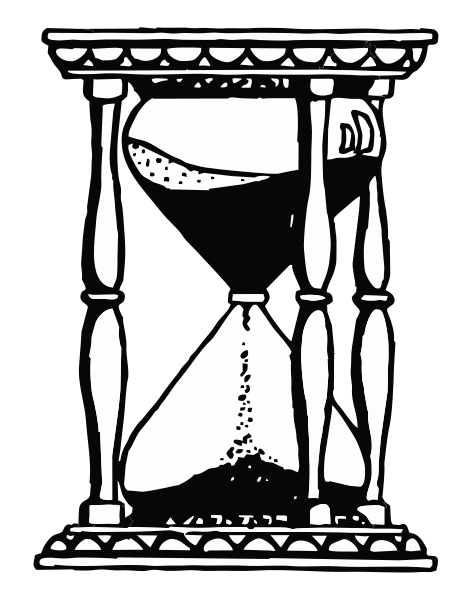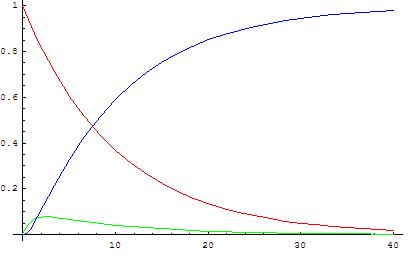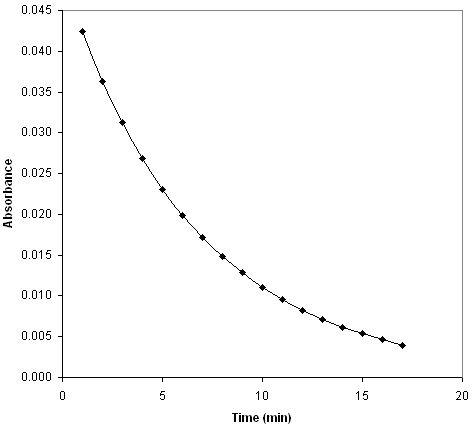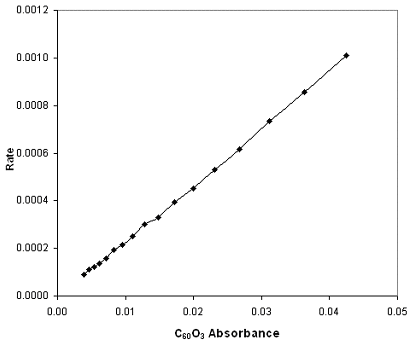## Rate Laws for Elementary Steps

The rate law for an elementary step is derived from the molecularity of that step.

### Learning Objectives

Write rate laws for elementary reactions, explaining how the order of the reaction relates to the reaction rate

### Key Takeaways

#### Key Points

• Elementary reactions sum to the overall reaction equation.
• Rate laws for elementary steps are derived from the molecularity of each step.
• Reaction mechanisms can agree with experimental data, but can never be proven for certain.

#### Key Terms

• unimolecular: Involving a single molecule.
• molecularity: Referring to the number of individual molecules involved in an elementary step of a reaction mechanism.
• bimolecular: Involving two molecules.
• elementary step: A single transformation in an overall reaction mechanism; can be unimolecular, bimolecular, or termolecular.

### Reaction Mechanisms

Every chemical reaction proceeds according to a reaction mechanism, which is a step-by-step description of what occurs during a reaction on the molecular level. Each step of the mechanism is known as an elementary process, which describes a single moment during a reaction in which molecules break and/or form new bonds. It is important to keep in mind that every reaction mechanism is simply a proposed version of what might be occurring at the molecular level; even if a mechanism agrees with experimental results, it is impossible to prove a reaction mechanism for certain. That being said, there are two strict requirements that must be fulfilled for a reaction mechanism to be valid. They are:

1. The sum of each elementary step in a reaction mechanism must yield the overall reaction equation.
2. The rate law of the rate-determining step must agree with the experimentally determined rate law.

The rate-determining step is the slowest step in a reaction mechanism. Because it is the slowest, it determines the rate of the overall reaction. This will be explored later in more detail.Change in concentration of chemicals over time: A plot of time versus concentration for two species in chemical equilibrium.

### Elementary Reactions and Their Rate Laws

Elementary reactions are classified in terms of their molecularity; that is, by how many molecules are involved in the given step. There are three possible molecularites:

1. unimolecular ($\text{A} \rightarrow \text{products}$)
2. bimolecular ($\text{A} + \text{B} \rightarrow \text{products}$)
3. termolecular ($\text{A} + \text{B} + \text{C} \rightarrow \text{products}$)

Termolecularity is not common due to the rarity of three molecules colliding at the same time, and in just the right way for reaction to occur.

The molecularity of the elementary step, and the reactants involved, will determine what the rate law will be for that particular step in the mechanism.Molecularity of elementary steps and corresponding rate laws: The molecularity of an elementary step in a reaction mechanism determines the form of its rate law.

### Example of Elementary Steps and Their Rate Laws

Consider the following reaction:

$2\;\text{NO}(\text{g})+\text{O}_2(\text{g})\rightarrow 2\;\text{NO}_2(\text{g})$

The overall equation suggests that two NO molecules collide with an oxygen molecule, forming NO2. However, because termolecular reactions are extremely rare, this reaction most likely consists of two or more elementary steps. Upon further analysis, the mechanism is given as:

$(1)\quad\quad 2\;\text{NO}(\text{g})\rightarrow \text{N}_2\text{O}_2(\text{g})\quad\quad \text{rate}=\text{k}_1[\text{NO}]^2$

$(2)\quad\quad \text{N}_2\text{O}_2(\text{g})+\text{O}_2(\text{g})\rightarrow 2\;\text{NO}_2(\text{g})\quad\quad \text{rate}=\text{k}_2[\text{N}_2\text{O}_2][\text{O}_2]$

Note that the two steps here add to the overall reaction equation, as the intermediate N2O2 cancels. However, we cannot simply add the rate laws of each elementary step in order to get the overall reaction rate. Determining the overall reaction rate from the reaction mechanism will be discussed in the next concept. For now, just keep in mind that the rate laws for each elementary step are determined by the molecularity of each step only.

## Rate-Determining Steps

The rate of a multi-step reaction is determined by the slowest elementary step, which is known as the rate-determining step.

### Learning Objectives

Describe the relationship between the rate determining step and the rate law for chemical reactions

### Key Takeaways

#### Key Points

• Chemists often write chemical equations for reactions as a single step, which shows only a reaction’s net result. However, most chemical reactions occur over a series of elementary reactions. The reaction mechanism is the step-by-step process by which reactants actually become products.
• The overall reaction rate depends almost entirely on the rate of the slowest step. If the first step is the slowest, and the entire reaction must wait for it, then it is the rate-determining step.

#### Key Terms

• rate-determining step: The slowest individual transformation in a reaction mechanism.

### Rate-Determining Steps

Chemists often write chemical equations for reactions as a single step that shows only the net result of a reaction. However, most chemical reactions occur over a series of elementary reactions. The complete sequence of these elementary steps is called a reaction mechanism. The reaction mechanism is the step-by-step process by which reactants actually become products. It is the “how” of the reaction, whereas the overall balanced equation shows only the “what” of the reaction. In kinetics, the rate of a reaction with several steps is determined by the slowest step, which is known as the rate-determining, or rate-limiting, step.

### Rate Laws and the Rate-Determining Step

Take the following example of a gas phase reaction:

$\text{CO} + \text{NO}_2 \rightarrow \text{CO}_2 + \text{NO}$

If this reaction occurred in a single step, its rate law would be:

$\text{r} = \text{k}[\text{NO}_2][\text{CO}]$

However, experiments show that the rate equation is:

$\text{r} = \text{k}[\text{NO}_2]^2$Hourglass analogy to the rate-determining step: The rate-determining step is like the narrowest point in the hourglass; it is the “bottleneck” point of the reaction that determines how quickly reactants can become products.

The fact that the experimentally-determined rate law does not match the rate law derived from the overall reaction equation suggests that the reaction occurs over multiple steps. Further, the experimental rate law is second-order, suggesting that the reaction rate is determined by a step in which two NO2 molecules react, and therefore the CO molecule must enter at another, faster step. A possible mechanism that explains the rate equation is:

$2\;\text{NO}_2 \rightarrow \text{NO}_3 + \text{NO}$ (slow step, rate-determining)

$\text{NO}_3 + \text{CO} \rightarrow \text{NO}_2 + \text{CO}_2$ (fast step)

Since the first step is the slowest, and the entire reaction must wait for it, it is the rate-determining step. We can picture the rate-determining step to be like the narrowest point in an hourglass; it is the “bottleneck” point of the reaction that determines how quickly reactants can become products.

If the first step in a mechanism is rate-determining, it is easy to find the rate law for the overall expression from the mechanism. If the second or a later step is rate-determining, determining the rate law is slightly more complicated. We will explore how to write that rate law later.

## Overall Reaction Rate Laws

Rate laws for reactions are affected by the position of the rate-determining step in the overall reaction mechanism.

### Learning Objectives

Combine elementary reaction rate constants to obtain equilibrium coefficients and construct overall reaction rate laws for reactions with both slow and fast initial steps

### Key Takeaways

#### Key Points

• In a reaction with a slow initial step, the rate law will simply be determined by the stoichiometry of the reactants.
• In a rate law with a fast initial step, no intermediates can appear in the overall rate law.

#### Key Terms

• rate-determining step: The slowest step in a chemical reaction that determines the rate of the overall reaction.

### Slow Step Followed by a Fast Step

As discussed in the previous concept, if the first step in a reaction mechanism is the slow, rate-determining step, then the overall rate law for the reaction is easy to write, and simply follows the stoichiometry of the initial step. For example, consider the following reaction:

$\text{H}_2(\text{g}) + 2\;\text{ICl}(\text{g})\rightarrow \text{I}_2(\text{g})+2\;\text{HCl}(\text{g})$

The proposed reaction mechanism is given as follows:

$(1)\;\text{slow reaction:}\quad \text{H}_2+\text{ICl}\rightarrow \text{HI}+\text{HCl}\quad\quad \text{rate}_1=\text{k}_1[\text{H}_2][\text{ICl}]$

$(2)\;\text{fast reaction:}\quad \text{HI}+\text{ICl}\rightarrow \text{I}_2+\text{HCl}\quad\quad \text{rate}_2=\text{k}_2[\text{HI}][\text{ICl}]$

Since the first step is the rate-determining step, the overall reaction rate for this reaction is given by this step: $\text{rate}=\text{k}[\text{H}_2][\text{ICl}]$. As it turns out, this rate law has been verified with experimental evidence.

### Fast Step Followed by a Slow Step

If the rate-determining step is not the first step in the reaction mechanism, the derivation of the rate law becomes slightly more complex. Consider the following reaction:

$2\;\text{NO}(\text{g})+\text{O}_2(\text{g})\rightarrow 2\;\text{NO}_2(\text{g})$

The proposed mechanism is given by:

$(1)\;\text{Step 1, fast:}\quad 2\;\text{NO}(\text{g})\rightleftharpoons \text{N}_2\text{O}_2(\text{g})\quad\quad \text{rate}_1=\text{k}_1[\text{NO}]^2$

$(2)\;\text{Step 2, slow:}\quad \text{N}_2\text{O}_2(\text{g})+\text{O}_2(\text{g})\rightarrow 2\;\text{NO}_2(\text{g})\quad\quad \text{rate}_2=\text{k}_2[\text{N}_2\text{O}_2][\text{O}_2]$

Step two is the slow, rate-determining step, so it might seem reasonable to assume that the rate law for this step should be the overall rate law for the reaction. However, this rate law contains N2O2, which is a reaction intermediate, and not a final product. The overall rate law cannot contain any such intermediates, because the rate law is determined by experiment only, and such intermediates are not observable. To get around this, we need to go back and consider the first step, which involves an equilibrium between NO and N2O2. At equilibrium, the rate of the forward reaction will equal the rate of the reverse reaction. We can write this as follows:

$\text{k}_1[\text{NO}]^2=\text{k}_{-1}[\text{N}_2\text{O}_2]$

Rearranging for [N2O2], we have:

$[\text{N}_2\text{O}_2]=\frac{\text{k}_1}{\text{k}_{-1}}[\text{NO}]^2$

We can now substitute this expression into the rate law for the second, rate-determining step. This yields the following:

$\text{rate}=\text{k}_2\left(\frac{\text{k}_1}{\text{k}_{-1}}[\text{NO}]^2\right)[\text{O}_2]=\text{k}[\text{NO}]^2[\text{O}_2]$

This overall rate law, which is second-order in NO and first-order in O2, has been confirmed experimentally.

Rate law for a mechanism with a fast initial step: How to determine the rate law for a mechanism with a fast initial step. Remember, the overall rate law must be determined by experiment. Therefore, the rate law must contain no reaction intermediates.

## Steady-State Approximation

The steady state approximation can be used to determine the overall rate law when the rate-determining step is unknown.

### Learning Objectives

Simplify overall rate laws using the steady state approximation for reactions with various or unknown rate-limiting steps, explainting the steady state approximation and when it is valid

### Key Takeaways

#### Key Points

• If the rate-limiting step is unknown, or if more than one step is rate-limiting, we assume that the rate of each elementary step is equal. The overall rate law will then be derived from the final step in the reaction mechanism.
• The steady state approximation assumes that the concentration of reaction intermediates remains constant throughout the reaction.
• The concentration of reaction intermediates is assumed to be steady because the intermediates are being produced as fast as they are consumed.

#### Key Terms

• steady state approximation: Sets the rate of change of a reaction intermediate in a reaction mechanism to zero.
• steady state: A situation in which all state variables are constant in spite of ongoing processes that strive to change them.

### The Steady State Approximation

In our discussion so far, we have assumed that every reaction proceeds according to a mechanism that is made up of elementary steps, and that there is always one elementary step in the mechanism that is the slowest. This slowest step determines the rate of the entire reaction, and as such, it is called the rate-determining step. We will now consider cases in which the rate-determining step is either unknown or when more than one step in the mechanism is slow, which affects the overall reaction rate. Both cases can be addressed by using what is known as the steady state approximation.

### Applying the Steady State Approximation

Recall our mechanism for the reaction of nitric oxide and oxygen:

$2\;\text{NO}(\text{g})\rightleftharpoons \text{N}_2\text{O}_2(\text{g})\quad\quad\quad\quad \text{Step 1}$

$\text{N}_2\text{O}_2(\text{g})+\text{O}_2(\text{g})\xrightarrow{\text{k}_2} 2\;\text{NO}_2(\text{g})\quad\quad \text{Step 2}$

Before, we assumed that the first step was fast, and that the second step was slow, thereby making it rate-determining. We will now proceed as if we had no such prior knowledge, and we do not know which, if either, of these steps is rate-determining. In such a case, we must assume that the reaction rate of each elementary step is equal, and the overall rate law for the reaction will be the final step in the mechanism, since this is the step that gives us our final products. In this case, the overall rate law will be:

$(1)\quad\quad \text{rate}=\text{k}_2[\text{N}_2\text{O}_2][\text{O}_2]$

However, this rate law contains a reaction intermediate, which is not permitted in this process. We need to write this rate law in terms of reactants only. In order to do so, we must assume that the state of the reaction intermediate, N2O2, remains steady, or constant, throughout the course of the reaction. The idea is analogous to a tub being filled with water while the drain is open. At a certain point, the flow of water into the tub will equal the flow of water out of the tub, so that the height of the water in the tub remains constant. In reality, however, water is flowing into and out of the tub at all times; the overall amount of water in the tub at any given time does not change. Our reaction intermediate, N2O2, is like the water in the tub, because it is being produced and consumed at equal rates. Therefore, we can rewrite our initial equilibrium step as the following combination of reverse reactions:

$2\;\text{NO}(\text{g})\xrightarrow{\text{k}_1} \text{N}_2\text{O}_2(\text{g})$

$2\;\text{NO}(\text{g})\xleftarrow{\text{k}_{-1}} \text{N}_2\text{O}_2(\text{g})$

Here, k1 and k-1 are the rate constants for the forward and reverse reactions, respectively. With the steady state assumption, we can write the following:

$\text{rate of formation of }\text{N}_2\text{O}_2=\text{rate of disappearance of }\text{N}_2\text{O}_2$

Now, both of these rates can be written as rate laws derived from our elementary steps. We can write them as follows:

$\text{k}_1[\text{NO}]^2=\text{k}_{-1}[\text{N}_2\text{O}_2]+\text{k}_2[\text{N}_2\text{O}_2][\text{O}_2]$

Note that the second side of the equation, which equals the rate of disappearance of our reaction intermediate N2O2, is written with two terms. The first term takes into account the disappearance of N2O2 in the reverse reaction of the initial step, and the second term takes into account the disappearance of N2O2 as a reactant in the second elementary step. We can rearrange this equation in terms of [N2O2]:

$\text{k}_1[\text{NO}]^2=[\text{N}_2\text{O}_2](\text{k}_{-1}+\text{k}_2[\text{O}_2])$

$\frac{\text{k}_1[\text{NO}]^2}{\text{k}_{-1}+\text{k}_2[\text{O}_2]}=[\text{N}_2\text{O}_2]$

Lastly, now that we have [N2O2] in terms of our reactants only, we can substitute this entire expression into our equation for the overall rate law (equation 1 above). This yields the following:

$\text{rate}=\frac{\text{k}_1\text{k}_2[\text{NO}]^2[\text{O}_2]}{\text{k}_{-1}+\text{k}_2[\text{O}_2]}$

Here we have our final rate law for the overall reaction. We had no knowledge of the rate-determining step, so we used the steady state approximation for our reaction intermediate, N2O2.Concentration of reactants, products, and intermediates vs. time: Reactant concentrations are indicated in red, product concentrations are indicated in blue, and intermediate concentrations are indicated in green. Notice that after their initial production, the concentration of the reaction intermediates remains relatively constant (slope of green curve is approximately zero) throughout the course of the reaction.

## Experimental Determination of Reaction Rates

Reaction rates can be determined experimentally by measuring the concentration of a reactant and/or product over time.

### Learning Objectives

Design experiments that use either chemical or physical properties to measure reaction rates

### Key Takeaways

#### Key Points

• Physical measurements can be performed on a system while it is reacting. These measurements have the advantage of not disturbing the system, and they can be taken during the reaction. Examples of these measurements are changes in volume, temperature, and absorption of a solution.
• Chemical methods of measurement yield concentrations directly. A small sample is extracted from the reacting mixture, and the reaction is halted by dilution, cooling the mixture long enough to measure concentrations, or adding another reagent to stop the reaction.
• For zero-order reactions, graph concentration vs. time to get a line with the slope -k. For first-order reactions, graph the logarithm of concentration vs. time to get a line with the slope -k. For second-order reactions, graph the reciprocal of concentration vs. time to get a line of slope -k.

#### Key Terms

• Beer’s law: Relates the attenuation of light to the properties of the material through which the light is traveling.
• absorbance: A logarithmic measure of the amount of light that is absorbed when passing through a substance.

### Experimental Methods for Measuring Reactions

In order to experimentally determine reaction rates, we need to measure the concentrations of reactants and/or products over the course of a chemical reaction. If we know the order of the reaction, we can plot the data and apply our integrated rate laws. For example, if the reaction is first-order, a plot of ln[A] versus t will yield a straight line with a slope of –k.

There are two main ways to measure the concentrations of reactions: by measuring the changes in an observable physical property, or by taking samples of the reaction solution and measuring concentration directly.

### Physical Measurements

Physical measurements can be performed on the system while it is reacting. These measurements have the advantage of not disturbing the reacting system, and they can usually be measured quickly. For example, if the total number of moles of gas changes during a gas reaction, the course of the reaction can be measured by monitoring the change in pressure at a constant volume. Other physical measurements include optical methods, such as measuring the change in light polarization, the change in the refractive index of the solution, or quite commonly, the change in the color of the solution, and therefore the absorption spectrum. Common electrical methods include changes in the conductivity of a solution, the electrical potential in a cell, and mass spectrometry. Other methods include thermal conductivity, viscosity, heats of reaction, and freezing points.

### Chemical Measurements

Chemical methods yield concentrations directly. A small sample is extracted from the reacting mixture, and the reaction is halted either by dilution, by cooling the mixture, or by adding another chemical reagent to stop the reaction. One disadvantage of this method is that removing a part of the reacting system, or adding an additional reagent to it, gradually changes it over time. Also, there is a time lag between when the sample is taken and when the reaction is measured, which makes the measurement less accurate.

### Using Beer’s Law to Measure Concentration Over Time

One oxidized form of buckminsterfullerene (C60) is C60O3. When a solution of C60O3 is warmed, the compounded decomposes, releasing O2 and creating C60O. The reaction is given by:

$\text{C}_{60}\text{O}_3\rightarrow \text{O}_2+\text{C}_{60}\text{O}$

The rate of this reaction can be monitored by measuring the absorbance of the solution. The absorbance is given by Beer’s law:

$\text{A}=\text{abC}$

In this equation, a is the absorptivity of a given molecules in solution, which is a constant that is dependent upon the physical properties of the molecule in question, b is the path length that travels through the solution, and C is the concentration of the solution. We’re interested in determining C.

By running the reaction of interest inside a spectrometer, the absorbance of the solution can be measured over time. The data can then be plotted.Oxidized buckminsterfullerene absorbance: The absorbance is proportional to the concentration of the C60O3 in solution, so observing the absorbance as a function of time is essentially the same as observing the concentration as a function of time.

By Beer’s law, the absorbance of the solution is directly proportional to the concentration of the C60O3 in solution, so observing the absorbance as a function of time is essentially the same as observing the concentration as a function of time. In this case, the rate law is given by:

$\text{rate}=\text{k}[\text{C}_{60}\text{O}_3]$

Therefore, a plot of the rate versus the absorbance will yield a straight line with a slope of k.Reaction rate vs. absorbance: The absorbance is directly proportional to the concentration, so this is simply a plot of the rate law, rate = k[C60O3], and the slope of the line is the rate constant, k.

### Plotting Integrated Rate Laws to Determine Reaction Rate

As discussed in a previous concept, plots derived from the integrated rate laws for various reaction orders can be used to determine the rate constant k. Recall that for zero-order reactions, a graph of [A] versus time will be a straight line with slope equal to –k. For first-order reactions, a graph of ln[A] versus time yields a straight line with a slope of –k, while for a second-order reaction, a plot of 1/[A] versus t yields a straight line with a slope of k.